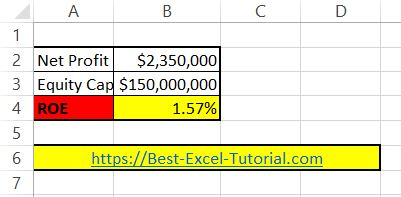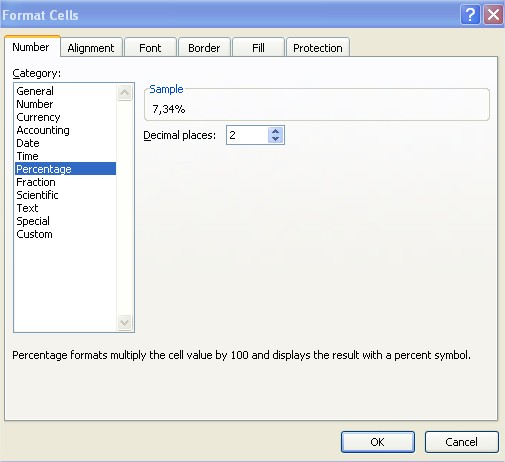#### How to calculate ROE?

In this lesson, you will learn how to calculate ROE in Excel.

What does ROE stand for?

ROE stands for return on equity. Return of equity is one of the most basic measures in business. ROE is a profitability indicator that is used to measure the efficiency of the company, regardless of the structure of its assets or extraordinary factors. The return on equity assists investors in determining the profit from their investment. ROE is a financial ratio that measures the efficiency of a company’s use of its shareholder’s equity to generate profit.

## ROE calculator

Let’s build the return on equity calculator in Excel. To calculate ROE in Excel, first you need some data. You need net profit and equity capital.

Copy and paste this ROE formula into cell B4: =B2/B3This formula will calculate the ROE for the data you place in cells B2 and B3 and is based on the roe equation formula:

ROE = Net Profit / Equity Capital

Remember to format ROE as Percentage. Click B4 cell > click CTRL + 1 keyboard shortcut > click Percentage with 2 decimal places.Note: The net income and shareholder’s equity figures should be for the same time period, such as a quarter or a year, to ensure an accurate comparison.